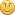## Formula for "Rate" of resources in schematics w/ multiple "Experimentation Prop"

Started by tiro_silex, Jan 02, 2022, 07:49 pm

#### tiro_silex

##### Jan 02, 2022, 07:49 pmLast Edit: Jan 02, 2022, 08:01 pm by tiro_silex
Hello,

On SWGAide's website, when opening a schematic, there's a list of Server Best resources for this schematic, ranked by a figure called "Rate". Calculating this rate is pretty straightforward when only a single "Experimentation Property" is selected. However, I would like to know how "Rate" is calculated whenever more than one and different properties are selected.

An example:

For Bivoli Tempari, one Experimentation Property is called "Experimental Flavor FL=66% OQ=33%". When only this is selected, the SB vegetable on server Finalizer  is Vapoo, with a rating of 713.30. So far so good.

When only selecting another property, "Experimental Nutritional Value PE=66% OQ=33%", Vapoo has a rating of 628.70. No problemo.

However, if we select both the properties mentioned above, a new Rate is calculated. I would perhaps expect this to be the average of the two figures given above, i.e. (713.30 + 628.70) / 2 = 671. However, the rate returned by SWGAide is 661.60. Link:

https://swgaide.com/schematics/view.php?server=163&schid=576

So my question is, how is this last Rate, with both Properties selected, calculated?

Also please note that in this case, the resource stats cap of Vegetables is 1-1000 for all stats, so it is not caps affecting the calculation here.

In this instance, if I go to Galaxy Harvester and look at the same schematic, server, resource and experimentation properties, it does in fact return a rate of 671, as I would expect. Link:

http://galaxyharvester.net/schematics.py/food_dish_bivoli_tempari

However, based on earlier anecdotal experience, also GH does not always return the expected average of the selected properties, but some other Rate all together.

I understand that such an aggregated Rate is entirely a theoretical measure, as in game, there is no one, overall rate depending on the resource's stats. However, I still find it interesting, because this calculation can impact on what we consider SB resources.

Can any of you please help shed some light on what is going on here? Thanks!EDIT: Also SWGCraft is showing a rate of 671 for the example above. Link:

http://swgcraft.org/dev/viewschematic.php?schem=6013

The more I think about it, it seems there must be a bug here, somewhere on SWGAide?

#### Mr-Miagi

##### Jan 05, 2022, 04:06 pm #1
Quote(resource_value / cap_of_schem_res_class * 1000) * (weight_exp / 100) * (resource_units / contributed_units)

Maths are explained here:  https://swgaide.com/swgaide_help_schematics_test_bench_en.html

#### tiro_silex

##### Jan 06, 2022, 08:35 pm #2
Thanks for your response, but this only explains how the rate is calculated within a single, experimentation property at a time:

Quote from: undefinedResults correspond to the merged experimental groups at The Laboratory, but just one per unique combination of required stats and weights.

My question is, the website SWGAide also caculates a different Rate if say two different unique combination of required stats and weights (i.e. Experimentation Properties) are selected. My question is how this rate is calculated? I am fairly sure such a Rate is not calculated in the desktop app.

#### Mr-Miagi

##### Jan 06, 2022, 11:14 pm #3
Quote from: tiro_silex on Jan 06, 2022, 08:35 pmThanks for your response, but this only explains how the rate is calculated within a single, experimentation property at a time:

Quote from: undefinedResults correspond to the merged experimental groups at The Laboratory, but just one per unique combination of required stats and weights.

My question is, the website SWGAide also caculates a different Rate if say two different unique combination of required stats and weights (i.e. Experimentation Properties) are selected. My question is how this rate is calculated? I am fairly sure such a Rate is not calculated in the desktop app.

The maths on both the site and the app are the same, less the resource quantities used.

#### tiro_silex

##### Jan 07, 2022, 02:26 pm #4
Maybe I'm missing something here, but what I'm talking about is only calculated on the site, not in the app...This is what I mean:
This is for Bivoli Tempari on Finalizer(642,7 + 841,3) / 2 = 742

...and NOT 766,4 as shown below:As opposed to what SWGCraft calculates when the exact same parameters are chosen: